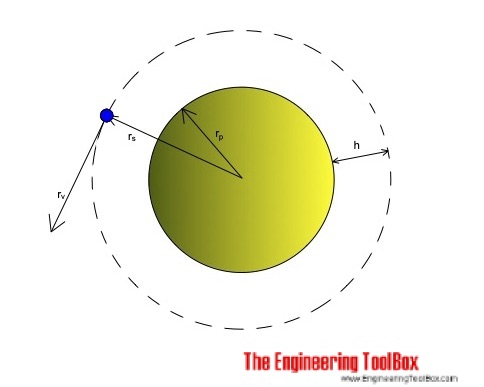Engineering ToolBox - Resources, Tools and Basic Information for Engineering and Design of Technical Applications!

# Satellites in Orbit

## Orbital and escape velocity for geostationary satellites.Orbital velocity of a satellite is at maximum at sea level and decreases with height.

Orbital velocity can be calculated as

vs = (g rp2 / rs)1/2                                (1)

where

vs = orbital velocity (m/s)

g = acceleration due to gravity (m/s2) (9.81 m/s2)

rp = radius planet (m) (earth: 6.37 106 m)

rs = radius satellite orbit (m)

Maximum velocity at sea level where radius planet equals radius orbit can be expressed as

vs_max = (g rp)1/2                                (1b)

Escape velocity where the satellite will leave its orbit and escape the planet gravity can be calculated as

vs_escape = (2 g rp)1/2                                (2)

Orbit periodic time can be expressed as

ts = 2 π (rs3 / (g rp2))1/2                         (3)

where

ts = orbit time (s)

Height of orbit can be calculated as

hs = rp ((g ts2 / (4 π2 rp))1/3 - 1)                     (4)

where

hs = height of orbit (m)

### Example - Earth bound Satellites

Maximum velocity at sea level:

vs_max = ((9.81 m/s2) (6.37 106 m))1/2

= 7905 m/s

= 7.9 km/h

Escape velocity at sea level:

vs_escape = (2 (9.81 m/s2) (6.37 106 m))1/2

= 11179 m/s

= 11.2 km/h

Height of the synchronous orbit for a geostationary satellite can be calculated by using (4) for an orbit period of 24 hours or 86400 s:

hs = (6.37 106 m) (((9.81 m/s2) (86400 s)2 / (4 π2 (6.37 106 m)))1/3 - 1)

= 35968 km

## Related Topics

• Miscellaneous - Engineering related topics like Beaufort Wind Scale, CE-marking, drawing standards and more.

## Engineering ToolBox - SketchUp Extension - Online 3D modeling!

Add standard and customized parametric components - like flange beams, lumbers, piping, stairs and more - to your Sketchup model with the Engineering ToolBox - SketchUp Extension - enabled for use with the amazing, fun and free SketchUp Make and SketchUp Pro .Add the Engineering ToolBox extension to your SketchUp from the SketchUp Pro Sketchup Extension Warehouse!

Translate

## Privacy

We don't collect information from our users. Only emails and answers are saved in our archive. Cookies are only used in the browser to improve user experience.

Some of our calculators and applications let you save application data to your local computer. These applications will - due to browser restrictions - send data between your browser and our server. We don't save this data.

## Citation

• Engineering ToolBox, (2019). Satellites in Orbit. [online] Available at: https://www.engineeringtoolbox.com/satellites-orbital-velocity-height-escape-d_2173.html [Accessed Day Mo. Year].

Modify access date.

. .

#### Scientific Online Calculator3 10